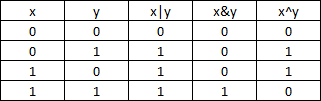# Bit wise operators in C:

These operators are used to perform bit operations. Decimal values are converted into binary values which are the sequence of bits and bit wise operators work on these bits.

• Bit wise operators in C language are & (bitwise AND), | (bitwise OR), ~ (bitwise NOT), ^ (XOR), << (left shift) and >> (right shift).

# Truth table for bit wise operation & Bit wise operators:# Below are the bit-wise operators and their name in C language.

1. & – Bitwise AND
2. | – Bitwise OR
3. ~ – Bitwise NOT
4. ^ – XOR
5. << – Left Shift
6. >> – Right Shift

Consider x=40 and y=80. Binary form of these values are given below.

x = 00101000
y=  01010000

All bit wise operations for x and y are given below.

1. x&y = 00000000 (binary) = 0 (decimal)
2. x|y = 01111000 (binary) = 120 (decimal)
3. ~x = 11111111111111111111111111 11111111111111111111111111111111010111 = -41 (decimal)
4. x^y = 01111000 (binary) = 120 (decimal)
5. x << 1 = 01010000 (binary) = 80 (decimal)
6. x >> 1 = 00010100 (binary) = 20 (decimal)

# Note:

• Bit wise NOT : Value of 40 in binary is 00000000000000000000000000000000 00000000000000000010100000000000. So, all 0’s are converted into 1’s in bit wise NOT operation.
• Bit wise left shift and right shift : In left shift operation “x << 1 “, 1 means that the bits will be left shifted by one place. If we use it as “x << 2 “,  then, it means that the bits will be left shifted by 2 places.

# Example program for bit wise operators in C:

In this example program, bit wise operations are performed as shown above and output is displayed in decimal format.

# Output:

 AND_opr value = 0 OR_opr value = 120 NOT_opr value = -41 XOR_opr value = 120 left_shift value = 80 right_shift value = 20

# Continue on types of C operators:

Click on each operator name below for detailed description and example programs.

 Types of Operators Description Arithmetic_operators These are used to perform mathematical calculations like addition, subtraction, multiplication, division and modulus Assignment_operators These are used to assign the values for the variables in C programs. Relational operators These operators are used to compare the value of two variables. Logical operators These operators are used to perform logical operations on the given two variables. Bit wise operators These operators are used to perform bit operations on given two variables. Conditional (ternary) operators Conditional operators return one value if condition is true and returns another value is condition is false. Increment/decrement operators These operators are used to either increase or decrease the value of the variable by one. Special operators &, *, sizeof( ) and ternary operators.